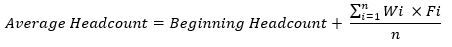top of page

# KPI Series: ProductivityIn this post, a method is introduced to calculate the productivity of the sales team. The purpose of this metric is to understand how much resource has been utilized to generate revenue over a specific period of time.

By definition, productivity is the output divided by the input (duh!). Simply, to calculate productivity per sales rep, we divide the total revenue (or output of any kind) over the number of sales reps for the targeted time period. In this case, the total revenue over a specific time period is clearly defined and can easily be measured. But what about the number of sales reps?

If the number of sales reps has been constant over that time period (congrats! Great employee retention performance!), we have the answer. But what if the sales team hiring and attrition rates are high and the average count fluctuates drastically? In that case, the average, beginning headcount or ending headcount won’t represent the number of sales reps for that specific time period and requires another solution.As a result, even though, sales rep count is not a continuous variable, it should be treated like one. The denominator, will have to account for all the changes during the specified time period – not simply an average. To create this metric, let’s consult the Modified Dietz Method that is used to measure the historical performance of an investment portfolio including all the inflows and outflows during the understudy period. According to this method, the return on an investment is gain or loss of the portfolio divided by the sum of the original value and weighted inflows/outflows:EV is the Ending Value

BV is the beginning Value

NF is the net cash flows (inflows minus outflows)

F is either the inflow or the outflow

W is the weight given to the cash flow to account for the timing of the inflow/outflow

In the productivity method presented in this case, we will only use the denominator from the modified Dietz method.

But first, let’s look at the weight (W) together. According to the time value of the money, the timing of the cash flow is important to the value of our portfolio. A dollar invested/received today has a higher value than a dollar invested/received a year from now. So, the weighting (W) in this model is there to capture the time value of the money. In a sense, the average value is the beginning value (BV) plus all the inflows (F) and minus all the outflows (F) where the timing of the flow has been factored in using the weight (W).

Now, how do we use the denominator in this productivity method? We need to take the beginning headcount and move it through the time by adding or deducting changes to/or from that. So, with this modified Dietz method our average headcount will be as follows:Fi is the flow of headcount. It’s positive for the count of new hires and negative for the number of terms in month of i.

Wi is the weight of month i that factors in the significance of month i contribution to productivity.

N is the number of months in the understudy period

The weight is defined under the assumption that the positive/negative contribution of a new hire/ termination to productivity in the month of January is 12 times more than December, when calculating productivity for a calendar year. So, both January new hires (+) and terms (-) should be weighted 12 and the weight should be counted down till December new hires and terms get 1.

That means, our productivity using the modified Dietz method can be defined as follows:Considerations:

• n can be a daily count, instead of monthly, if necessary.

• Weighting system can be adjusted to the nature of the business.

• For new hires the contribution can be included after training or a ramping up period. If it takes 6 months for a new rep to become productive, we might want to exclude them from the sales count for the first 6 months, even though they’re still taking resources.

• The productivity might be different for reps with different experience or tenure level. As a result, sub categories or clusters can be introduced within the sales team to compare apple to apple and not to let irrelevant categories dilute the result. The industry, market, geography, line of business that the rep is operating in can also define the clusters and other sub categories.

• The sales cycle can also be accounted for in the productivity calculation. For example, if you’re calculating the productivity for a calendar year and your average sales cycle is 60 days, you should divide the revenue of that calendar year by the average headcount calculated from the beginning headcount in November of the previous year to the end headcount of October of the understudy year.

bottom of page